Disclaimer: This is an example of a student written essay.
Click here for sample essays written by our professional writers.

Any scientific information contained within this essay should not be treated as fact, this content is to be used for educational purposes only and may contain factual inaccuracies or be out of date.

# Pattern Recognition and Classification Theory

 ✅ Paper Type: Free Essay ✅ Subject: Engineering ✅ Wordcount: 1454 words ✅ Published: 31st Jul 2017

Assignment 2 of Pattern recognition should contain the classification theory. The topics should cover:

1. Introduction to Pattern Recognition, including; a) The concept of pattern recognition and its applications. b) Basic steps of a typical pattern recognition task. c) Popular techniques used in these steps. d) Various application areas of pattern recognition research.
2. Bayesian classification rule, prior, posterior, loss function, risk, and minimum error rate classification.
3. Discriminant functions, Normal densities and application of Bayesian rule to normal densities with 3 different cases of variances and covariance matrices discussed in book.

As the name indicates, the pattern recognition is the classification of a pattern to one of the pre-specified classes. The process of understanding or recognizing the patterns by taking the raw data from a sensor, convert that raw data into some meta data by pre-processing that raw data, producing segments of the data through some sort of segmentation process and the pass those segment through some feature extractor which lead the purification of the raw data to be understood by the Classifier. Based on the feature extracted will classify it to a certain class which is already defined by the decision boundary. The decision boundary is obtained from a series of training data and the cost related to it.

In short, the process of identifying object or pattern into some sort of classes based on some features which are been described by the decision rule. A simple example for it the identification of the seabass and salmon fish passing through a conveyer belt. Certain features like the height, width and lightness can be used to develop a decision boundary and put any fish into its respected class (sea bass or salmon).

It is the analysis of in what way the machines observe the environment, come to know about the different patterns and make a rational decision about the class of the patterns.

A typical pattern recognition system consists of the following components:

1. Physical Environment
2. Data Acquisition/Sensing
3. Pre-Processing
4. Feature Extraction
5. Features
6. Classification
7. Post-Processing
8. Decision Making

The above mentioned components are given in the Figure 1.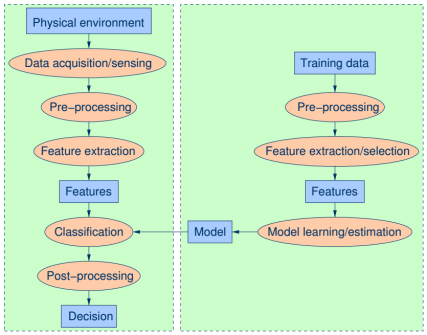Figure 1 Components of a Pattern Recognition System.

1. How to overcome the insufficiency of vector space?
2. Numerous amount of training data.
3. Anonymous distributions of classes.
4. Unidentified problem complexity.
5. Generalization problems.
6. Evaluation problems.

Given below are few of the pattern recognition potential research areas:

• Adaptive signal processing
• Machine learning
• Artificial neural networks
• Robotics and vision
• Cognitive sciences
• Mathematical statistics
• Nonlinear optimization
• Exploratory data analysis
• Fuzzy and genetic systems
• Detection and estimation theory
• Formal languages
• Structural modeling
• Biological cybernetics
• Computational neuroscience

Pattern recognition has outnumbered amount of applications, some of which are as follows:

• Image processing
• Computer vision
• Speech recognition
• Multimodal interfaces
• Automated target recognition
• Optical character recognition
• Seismic analysis
• Man and machine diagnostics
• Fingerprint identification
• Industrial inspection
• Financial forecast
• Medical diagnosis
• ECG signal analysis

Given below are the fundamental steps involved in pattern recognition:

Sensing: The pattern recognition systems require a sensor at the input in order to take raw data from the environment into the system.

Segmentation: It is done after the pre-processing step. In some systems this is the pre-processing step used for converting the raw data into some sorted data for the feature extraction.

Feature Extraction: Some specific parameters of the pattern are measured in this step like length in the fish example.

Classification: The patterns are then classified through some sort of classifiers like Bayesian Classifier. Classification is done for putting the pattern into a specific class or category e.g. sea bass or salmon.

Post Processing: This step is done for further improvement of the performance.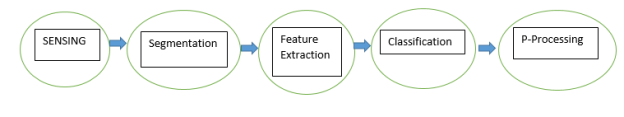Figure 2 Steps involved in Pattern Recognition.

Classification techniques:

Bayes classifier, HMM, Kth Nearest Neighbor (KNN), Artificial Neural Network (ANN), Support Vector Machines (SVM), Training (parameter finding) & testing (decoding) etc.

Data representation techniques:

The compacting technique is used for improving the characteristics features of data using various transformation methods like the Fourier Transform method, WT etc.

Dimensionality reduction:

Reduce the data dimensions by removing the mutually correlated features which results in the reduction of the common information to produce a set of nearly real informative parameters.

e.g. Principle Component Analysis, Linear Discriminant Analysis etc.

Transformations:

Various transformation techniques are also used like Fourier Transforms, Fast Fourier Transform etc.

The following are the potential research areas in the field of pattern recognition:

• Adaptive signal processing
• Machine learning
• Artificial neural networks
• Robotics and vision
• Cognitive sciences
• Mathematical statistics
• Nonlinear optimization
• Exploratory data analysis
• Fuzzy and genetic systems
• Detection and estimation theory
• Formal languages
• Structural modeling
• Biological cybernetics
• Computational neuroscience

The probability of a state of nature that show how likely is that, that particular state of nature would occur. For example, in the fish example it is given that the prior of the salmon is 0.85. This mean that salmon is 85% more likely to appear than the sea bass. If number of classes are c, then: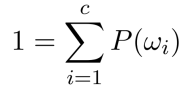It is the probability of a specific state of nature given that observables have occurred. Mathematically,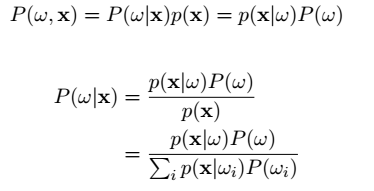Notice that,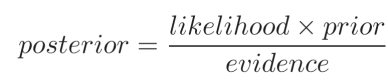It shows the cost related to each wrong action or decision we take. Mathematically,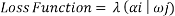The zero-one is the most commonly used loss function. It assigns zero on no loss in case of correct decision while in case of incorrect decision, it takes a uniform unit loss. Mathematically,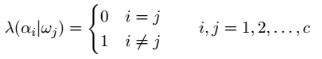The expected loss is also called as conditional risk. It is defined as the summation of the product of loss occurred from each decision to its posterior probability. Mathematically: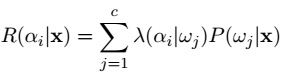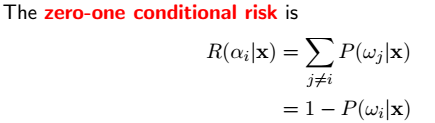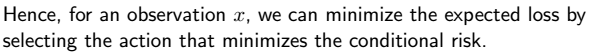Overall risk is given by: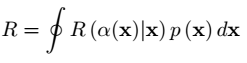From above equation we come to know that by selecting only those action Î± (.) that minimize the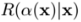for all values of x will minimize the overall risk which is directly associated with the error thus minimize the error rate.

## Cite This Work

To export a reference to this article please select a referencing stye below:

View all

## DMCA / Removal Request

If you are the original writer of this essay and no longer wish to have your work published on UKEssays.com then please:

Related Services

Related Lectures

Study for free with our range of university lectures!Freelance Writing Jobs

Looking for a flexible role?
Do you have a 2:1 degree or higher?

Study Resources

Free resources to assist you with your university studies!